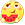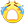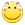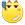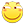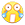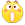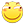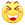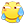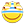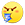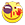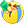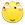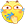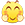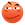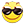# 将评论邮件提醒插件加入每日发送量阈值的功能

``````    /**
* 频数限制
*
* @access public
* @return bool
*/
public static function mailLimit() {
\$db= Typecho_Db::get();
\$query= \$db->select('date','num')->from('table.maillimiter');
\$result = \$db->fetchAll(\$query);
\$num = \$result['num'];

//判断是否为当日
if ( date('Ymd',\$result['date']) != date('Ymd',time()) ){
//如果不是当日，那就更新一个当日的时间戳，并让计数器归零
\$time = time();
\$num = 0;
\$updateToday = \$db->update('table.maillimiter')->rows(array('date'=> \$time ,"num" => \$num ))->where('jikey=?',1);
\$updateTodayRows= \$db->query(\$updateToday);
}

//判断是否限额
if (\$num < 200){
//未到上限，数据递增
\$num ++;
\$updateToday = \$db->update('table.maillimiter')->rows(array("num" => \$num ))->where('jikey=?',1);
\$updateTodayRows= \$db->query(\$updateToday);
return 1;
} else {
//已到上限，返回“否”
return 0;
}
}``````

（不要在意默认值的问题，因为这张表是拿我另外一张干别的用的表修改来的。）

``````        if (self::mailLimit()){
\$mail->send();
} else {
//此处可以加一个Server酱什么的，做一个吿薪处理
}``````

1. 暂无评论 / 0 Comment

😉